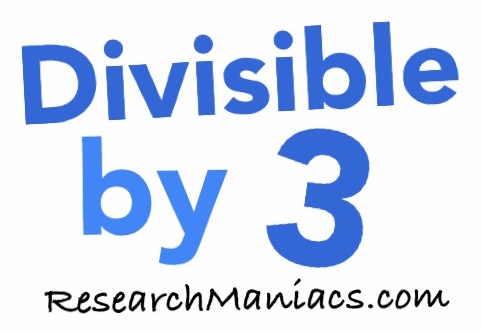Is 46 divisible by 3?

 Is 46 divisible by 3? In other words, if you divide 46 by 3, will you get a whole number with no remainder? Of course, you could use a calculator to find out if 46 is divisible by 3, but what fun would that be? To find out if 46 is divisible by 3, we will add up the digits that make 46 as follows: 4 + 6 = 10 We know that if the sum of the numbers that make up 46 is divisible by 3, then 46 is divisible by 3. Since the sum of the digits in 46 is not divisible by 3, 46 is also NOT divisible by 3. Thus, the answer to the question "Is 46 divisible by 3?" is as follows: No Note: If you divide 46 by 3, you get 15.33 which is not a whole number. Is 47 divisible by 3? Can you do it on your own now? If not, go here for the next number on our list.Divisible by 3? Do you need to check another number? Enter a number below to see if it is divisible by 3.